# Analytic number theory

The motivation for studying the zeros of the zeta-function is the precise relationship between the zeros of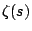and the errot term in the prime number theorem. Define the Von Mangoldt function by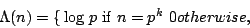where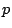is a prime number. Then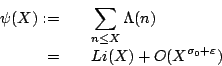if and only ifdoes not vanish for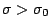. There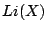is the logarithmic integral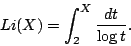In particular, the best possible error term in the prime number theorem is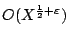, which is equivalent to the Riemann Hypothesis.

Similarly, the Generalized Riemann Hypothesis is equivalent to the best possible error term for the counting function of primes in arithmetic progressions.

Riemann Hypotheses for other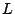-functions have not yet been shown to be strongly connected to the distribution of the prime numbers.

Back to the main index for The Riemann Hypothesis.